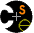CSE 378 Spring 2010HomeAdministrativeAcademic MisconductSyllabusHomeworkHomework 0 "Due" 4/13Homework 1 (sol'n)Homework 2    (Quicksort solution,   Smash solution)Homework 3 (sol'n)Homework 4 (doc, soln) Due 6/2 LabsLab 1 SW Due 4/16Lab 1 HW Due 4/16Lab 2 SW Due 4/30Lab 2 HW Due 4/30Lab 3 Due 5/14Lab 4 SW Due 6/2Lab 4 HW Due 6/2 ResourcesLecturesExamsWikiLab InfoGreen Sheet (PDF)Green Sheet MagicMIPS Resources CommunicationsDiscussion BoardMail List ArchivesTurninGradeBook Anonymous FeedbackFeedback Form# CSE378 Spring 2010 Homework 2

## Due April 28 at 5pm.

You may use two late days on this assignment.

The goal of this assignment is to learn about MIPS functions, calling conventions, and stack layout. There are two parts.

## Part A: Implementing Quicksort

For this part, you'll write your old friend, Quicksort - a recursive, average-case O(NlogN) sorting routine - in assembly. Here's some pseudo-code for Quicksort, to sort an array of integers in non-decreasing order:
```void quicksort (integer A[], integer p, integer r) begin
integer q;
if p < r then
q := partition(A, p, r)
quicksort(A, p, q)
quicksort(A, q+1, r)
end if
end quicksort

integer partition (integer A[], integer p, integer q) begin
integer pivot := A[p]
integer i := p-1
integer j := q+1
while (true)
repeat j := j-1 until (A[j] <= pivot)
repeat i := i+1 until (A[i] >= pivot)
if i < j then
swap(A, i, j)
else
return j
end if
end loop
end partition

void swap (integer A[], integer i, integer j) begin
integer temp := A[i]
A[i] := A[j]
A[j] := temp
end swap
```
The template calls Quicksort on an array of signed integers using the function signature in the pseudocode. We provide the swap function. You don't have to follow the pseudocode exactly as long as you implement Quicksort.

We will test on multiple arrays with interesting values. You may want to modify the array in the template to try other values.

### What you should do for part A:

2. Modify the parts of the code marked "TODO".
3. Load your program into SPIM and execute it. If your code works, the sorted array will be printed.

## Part B: Smashing the Stack

Now you'll use your knowledge of MIPS stack layout to "take over" a program. You will write a function in the MIPS assembly language that smashes the program's stack frame and verify it in SPIM.

### Exploiting a Vulnerable Program's Stack

This simple assembly program reads in a student's NetID, verifies that the NetID is in the correct format, and returns the class grade of the student. The problem with this program is that the local, stack-allocated buffer used for holding the NetID (inside the string_sanitize() function) is of fixed size, and the replace_spaces() function does not check that the destination buffer is large enough to hold what's copied into it. If the NetID is too big for the destination buffer, replace_spaces() will just keep writing past the end and overwrite whatever happens to be adjacent in memory.

You will write a function, attack_string(), to modify the netid_buffer so that a student is automatically awarded with a grade of A+ whenever the professor attempts to reduce his/her grade to a C-. To accomplish this, the netid_buffer must be changed such that when the program executes, the stack frame of validate_netid() gets smashed and the return address of validate_netid() gets overwritten. You need to inject the address of the automatic_a_plus() function on the stack so that when validate_netid() returns, it transfers the flow of control to the automatic_a_plus() function and runs the code in automatic_a_plus().

You need to change only one part of the program, marked with the word "TODO" near the bottom of the file. All other source code should remain intact.

### Sample Run

The output of this unmodified code looks like this:
```Accessing student record...
jnelson is a valid NetID.
-- Connection to Student Records terminated --
```
After you have implemented the attack_string() function, the program output should look like this:
```Accessing student record...
jnelson is a valid NetID.
-- Connection to Student Records terminated --
```

It may be helpful to sketch out the stack layout and size at the time that the string_sanitize() function is called, so that you know what value on the stack you have to smash.

You will have to consider endian-ness issues when crafting your value for input_buffer.

### What you should do for part B:Computer Science & Engineering University of Washington Box 352350 Seattle, WA  98195-2350 (206) 543-1695 voice, (206) 543-2969 FAX [comments to CSE 378 TAs]